# An efficient 3D topology optimization code written in Matlab

## Abstract

This paper presents an efficient and compact Matlab code to solve three-dimensional topology optimization problems. The 169 lines comprising this code include finite element analysis, sensitivity analysis, density filter, optimality criterion optimizer, and display of results. The basic code solves minimum compliance problems. A systematic approach is presented to easily modify the definition of supports and external loads. The paper also includes instructions to define multiple load cases, active and passive elements, continuation strategy, synthesis of compliant mechanisms, and heat conduction problems, as well as the theoretical and numerical elements to implement general non-linear programming strategies such as SQP and MMA. The code is intended for students and newcomers in the topology optimization. The complete code is provided in Appendix C and it can be downloaded from http://top3dapp.com.

## Introduction

Topology optimization is a computational material distribution method for synthesizing structures without any preconceived shape. This freedom provides topology optimization with the ability to find innovative, high-performance structural layouts, which has attracted the interest of applied mathematicians and engineering designers. From the work of Lucien Schmit in the 1960s (Schmit 1960)—who recognized the potential of combining optimization methods with finite-element analysis for structural design—and the seminal paper by Bendsøe and Kikuchi (1988), there have been more than eleven thousand journal publications in this area (Compendex list as of September 2013), several reference books (Hassani and Hinton 1998; Bendsøe and Sigmund 2003; Christensen and Klarbring 2009), and a number of readily available educational computer tools for Matlab and other platforms. Some examples of such tools include the topology optimization program by Liu et al. (2005) for Femlab, the shape optimization program by Allaire and Pantz (2006) for FreeFem++, the open source topology optimization program ToPy by Hunter (2009) for Python, and the 99-line program for Michell-like truss structures by Sokół (2011) for Mathematica.

For Matlab, Sigmund (2001) introduced the 99-line program for two-dimensional topology optimization. This program uses stiffness matrix assembly and filtering via nested loops, which makes the code readable and well-organized but also makes it slow when solving larger problems. Andreassen et al. (2011) presented the 88-line program with improved assembly and filtering strategies. When compared to the 99-line code in a benchmark problem with 7500 elements, the 88-line code is two orders of magnitude faster. From the same research group, Aage et al. (2013) introduced TopOpt, the first topology optimization App for hand-held devices.

Also for Matlab, Wang et al. (2004) introduced the 199-line program TOPLSM making use of the level-set method. Challis (2010) also used the level-set method but with discrete variables in a 129-line program. Suresh (2010) presented a 199-line program ParetoOptimalTracing that traces the Pareto front for different volume fractions using topological sensitivities. More recently, Talischi et al. (2012a, b) introduced PolyMesher and PolyTop for density-based topology optimization using polygonal finite elements. The use of polygonal elements makes these programs suitable for arbitrary non-Cartesian design domains in two dimensions.

One of the few contributions to three-dimensional Matlab programs is presented by Zhou and Wang (2005). This code, referred to as the 177-line program, is a successor to the 99-line program by Sigmund (2001) that inherits and amplifies the same drawbacks. Our paper presents a 169-line program referred to as top3d that incorporates efficient strategies for three-dimensional topology optimization. This program can be effectively used in personal computers to generate structures of substantial size. This paper explains the use of top3d in minimum compliance, compliant mechanism, and heat conduction topology optimization problems.

The rest of this paper is organized as follows. Section 2 briefly reviews theoretical aspects in topology optimization with focus on the density-based approach. Section 3 introduces 3D finite element analysis and its numerical implementation. Section 4 presents the formulation of three typical topology optimization problems, namely, minimum compliance, compliant mechanism, and heat conduction. Section 5 discusses the optimization methods and their implementation in the code. Section 6 shows the numerical implementation procedures and results of three different topology optimization problems, several extensions of the top3d code, and multiple alternative implementations. Finally, Section 7, offers some closing thoughts. The top3d code is provided in Appendix C and can also be downloaded for free from the website: http://top3dapp.com.

## Theoretical background

### Problem definition and ill-posedness

A topology optimization problem can be defined as a binary programming problem in which the objective is to find the distribution of material in a prescribed area or volume referred to as the design domain. A classical formulation, referred to as the binary compliance problem, is to find the “black and white” layout (i.e., solids and voids) that minimizes the work done by external forces (or compliance) subject to a volume constraint.

The binary compliance problem is known to be ill-posed (Kohn and Strang 1986a, b, c). In particular, it is possible to obtain a non-convergent sequence of feasible black-and-white designs that monotonically reduce the structure’s compliance. As an illustration, assume that a design has one single hole. Then, it is possible to find an improved solution with the same mass and lower compliance when this hole is replaced by two smaller holes. Improved solutions can be successively found by increasing the number of holes and reducing their size. The design will progress towards a chattering design within infinite number of holes of infinitesimal size. That makes the compliance problem unbounded and, therefore, ill-posed.

One alternative to make the compliance problem well-posed is to control the perimeter of the structure (Haber and Jog 1996; Jog 2002). This method effectively avoids chattering configurations, but its implementation is not free of complications. It has been reported that the addition of a perimeter constraint creates fluctuations during the iterative optimization process so internal loops need to be incorporated (Duysinx 1997) Op. cit. (Bendsøe and Sigmund 2003). Also, small variations in the parameters of the algorithm lead to dramatic changes in the final layout (Jog 2002).

### Homogenization method

Another alternative is to relax the binary condition and include intermediate material densities in the problem formulation. In this way, the chattering configurations become part of the problem statement by assuming a periodically perforated microstructure. The mechanical properties of the material are determined using the homogenization theory. This method is referred to as the homogenization method for topology optimization (Bendsøe 1995; Allaire 2001). The main drawback of this approach is that the optimal microstructure, which is required in the derivation of the relaxed problem, is not always known. This can be alleviated by restricting the method to a subclass of microstructures, possibly suboptimal but fully explicit. This approach, referred to as partial relaxation, has been utilized by many authors including Bendsøe and Kikuchi (1988), Allaire and Kohn (1993), Allaire et al. (2004), and references therein.

An additional problem with the homogenization methods is the manufacturability of the optimized structure. The “gray” areas found in the final designs contain microscopic length-scale holes that are difficult or impossible to fabricate. However, this problem can be mitigated with penalization strategies. One approach is to post-process the partially relaxed optimum and force the intermediate densities to take black or white values (Allaire et al. 1996). This a posteriori procedure results in binary designs, but it is purely numerical and mesh dependent. Other approach is to impose a priori restrictions on the microstructure that implicitly lead to black-and-white designs (Bendsøe 1995). Even though penalization methods have shown to be effective in avoiding or mitigating intermediate densities, they revert the problem back to the original ill-possedness with respect to mesh refinement.

### Density-based approach

An alternative that avoids the application of homogenization theory is to relax the binary problem using a continuous density value with no microstructure. In this method, referred to as the density-based approach, the material distribution problem is parametrized by the material density distribution. In a discretized design domain, the mechanical properties of the material element, i.e., the stiffness tensor, are determined using a power-law interpolation function between void and solid (Bendsøe 1989; Mlejnek 1992). The power law may implicitly penalize intermediate density values driving the structure towards a black-and-white configuration. This penalization procedure is usually referred to as the Solid Isotropic Material with Penalization (SIMP) method (Zhou and Rozvany 1991). The SIMP method does not solve the problem’s ill-possedness, but it is simpler than other penalization methods.

The SIMP method is based on a heuristic relation between (relative) element density x i and element Young’s modulus E i given by

$$\text E_{i} = \text E_{i}(x_{i}) = {x_{i}^{p}} \text E_{0}, \quad x_{i} \in ]0,1],$$
(1)

where E0 is the elastic modulus of the solid material and p is the penalization power (p > 1). A modified SIMP approach is given by

$$\text E_{i} = \text E_{i}(x_{i}) = \text E_{\min} + {x_{i}^{p}} (\text E_{0} - \text E_{\min}), \quad x_{i} \in [0,1],$$
(2)

where Emin is the elastic modulus of the void material, which is non-zero to avoid singularity of the finite element stiffness matrix. The modified SIMP approach, as (2), offers a number of advantages over the classical SIMP formulation, as shown in (1), including the independency between the minimum value of the material’s elastic modulus and the penalization power (Sigmund 2007).

However, topology optimization methods are likely to encounter numerical difficulties such as mesh-dependency, checkerboard patterns, and local minima (Bendsøe and Sigmund 2003). In order to mitigate such issues, researchers have proposed the use of regularization techniques (Sigmund and Peterson 1998). One of the most common approaches is the use of density filters (Bruns and Tortorelli 2001). A basic filter density function is defined as

$$\tilde{\mathrm{x}}_{i} = \frac {\sum_{j \in N_{i}} H_{ij} v_{j} x_{j}} {\sum_{j \in N_{i}} H_{ij} v_{j}},$$
(3)

where N i is the neighborhood of an element x i with volume v i , and H i j is a weight factor. The neighborhood is defined as

$$N_{i} = \left \{j : \text{dist}(i,j) \leqslant R \right \},$$
(4)

where the operator dist(i, j) is the distance between the center of element i and the center of element j, and R is the size of the neighborhood or filter size. The weight factor H i j may be defined as a function of the distance between neighboring elements, for example

$$H_{ij} = R - \text{dist}(i,j),$$
(5)

where jN i . The filtered density $$\tilde {x_{i}}$$ defines a modified (physical) density field that is now incorporated in the topology optimization formulation and the SIMP model as

$$\text E_{i}(\tilde{\mathrm{x}}_{i}) = \text E_{\min} + \tilde{\mathrm{x}}_{i}^{p} (\text E_{0} - \text E_{\min}), \quad \tilde{\mathrm{x}}_{i} \in [0,1].$$
(6)

The regularized SIMP interpolation formula defined by (6) used in this work.

## Finite element analysis

### Equilibrium equation

Following the regularized SIMP method given by (6) and the generalized Hooke’s law, the three-dimensional constitutive matrix for an isotropic element i is interpolated from void to solid as

$$\mathbf C_{i}(\tilde{\mathrm{x}}_{i}) = \text E_{i}(\tilde{\mathrm{x}}_{i}) \mathbf{C}^{0}_{i}, \quad \tilde{\mathrm{x}}_{i} \in [0,1],$$
(7)

where $$\mathbf {C}^{0}_{i}$$ is the constitutive matrix with unit Young’s modulus. The unit constitutive matrix is given by

$$\begin{array}{@{}rcl@{}} &&\mathbf{C}^{0}_{i} =\frac {1} {(1+\nu)(1-2\nu)} \times \\ && \left[\begin{array}{cccccccccc} 1 -\nu & \nu & \nu & 0 & 0 & 0 \\ \nu & 1-\nu & \nu & 0 & 0 & 0 \\ \nu & \nu & 1-\nu & 0 & 0 & 0 \\ 0 & 0 & 0 & (1-2\nu) \slash 2 & 0 & 0 \\ 0 & 0 & 0 & 0 & (1-2\nu) \slash 2 & 0 \\ 0 & 0 & 0 & 0 & 0 & (1-2\nu) \slash 2\\ \end{array}\right], \end{array}$$
(8)

where ν is the Poisson’s ratio of the isotropic material. Using the finite element method, the elastic solid element stiffness matrix is the volume integral of the elements constitutive matrix C i (x͂ i ) and the strain–displacement matrix B in the form of

$$\mathbf k_{i}(\tilde{\mathrm{x}}_{i}) = \int_{-1}^{+1} \int_{-1}^{+1} \int_{-1}^{+1} \mathbf B^{\mathrm{T}} \mathbf C_{i} (\tilde{\mathrm{x}}_{i}) \mathbf B d\xi_{1} d\xi_{2} d\xi_{3},$$
(9)

where ξ e (e = 1,…,3) are the natural coordinates as shown in Fig. 1, and the hexahedron coordinates of the corners are shown in Table 1. The strain–displacement matrix B relates the strain 𝜖 and the nodal displacement u, 𝜖 = Bu. Using the SIMP method, the element stiffness matrix is interpolated as

$$\mathbf k_{i}(\tilde{\mathrm{x}}_{i}) = \text E_{i}(\tilde{\mathrm{x}}_{i}) \mathbf{k}^{0}_{i},$$
(10)

where

$$\mathbf{k}^{0}_{i} = {\int}_{-1}^{+1} {\int}_{-1}^{+1} {\int}_{-1}^{+1} \mathbf B^{\text{T}} \mathbf C^{0} \mathbf B d\xi_{1} d\xi_{2} d\xi_{3}.$$
(11)Fig. 1

Replacing values in (11), the 24 × 24 element stiffness matrix $$\mathbf {k}^{0}_{i}$$ for an eight-node hexahedral element is

$$\mathbf{k}^{0}_{i} = \frac{1}{(\nu + 1)(1-2\nu)} \left[\begin{array}{llllllll} \mathbf{k}_{1} & \mathbf{k}_{2} & \mathbf{k}_{3} & \mathbf{k}_{4} \\ {\mathbf{k}}^{\text{{T}}}_{2} & \mathbf{k}_{5} & \mathbf{k}_{6} & {\mathbf{k}}^{\text{{T}}}_{4} \\ {\mathbf{k}}^{\text{{T}}}_{3} & \mathbf{k}_{6} & {\mathbf{k}}^{\text{{T}}}_{5} & {\mathbf{k}}^{\text{{T}}}_{2} \\ \mathbf{k}_{4} & \mathbf{k}_{3} & \mathbf{k}_{2} & {\mathbf{k}}^{\text{{T}}}_{1} \end{array}\right]$$
(12)

where k m (m = 1,…,6) are 6 × 6 symmetric matrices (see Appendix A). One can also verify that $$\mathbf{k}^{0}_{i}$$ is positive definite. The global stiffness matrix K is obtained by the assembly of element-level counterparts k i ,

$$\mathbf K(\tilde{\mathbf{x}}) = \mathcal A_{i=1}^{n} \mathbf k_{i}(\tilde{\mathrm{x}}_{i}) = \mathcal A_{i=1}^{n} {\text E}_{i}(\tilde{\mathrm{x}}_{i}) \mathbf{k}^{0}_{i},$$
(13)

where n is the total number of elements. Using the global versions of the element stiffness matrices K i and $$\mathbf {k}^{0}_{i}$$, (13) is expressed as

$$\mathbf K(\tilde{\mathbf{x}}) = {\sum}_{i=1}^{n} \mathbf{K}_{i}(\tilde{\mathrm{x}}_{i}) = {\sum}_{i=1}^{n} {\text E}_{i}(\tilde{\mathrm{x}}_{i}) \mathbf{K}^{0}_{i}.$$
(14)

where $$\mathbf {k}^{0}_{i}$$ is a constant matrix. Using the interpolation function defined in (6), one finally observes that

$$\mathbf K(\tilde{\mathbf{x}}) = {\sum}_{i=1}^{n} \left [ {\text E}_{{\text {min}}} + \tilde{\mathrm{x}}_{i}^{p}(\text E_{0} - \text E_{\text {min}}) \right ] \mathbf{K}^{0}_{i}.$$
(15)

Finally, the nodal displacements vector U(x͂) is the solution of the equilibrium equation

$$\mathbf{K}(\tilde{\mathbf{x}}) \mathbf{U}(\tilde{\mathbf{x}}) = \mathbf{F},$$
(16)

where F is the vector of nodal forces and it is independent of the physical densities . For brevity of notation, we omitted the dependence of physical densities on the design variables x, = (x).

### Numerical implementation

Consider the discretized prismatic structure in Fig. 2 composed of eight eight-noded cubic elements. The nodes identified with a number (node ID) ordered column-wise up-to-bottom, left-to-right, and back-to-front. The position of each node is defined with respect to Cartesian coordinate system with origin at the left-bottom-back corner.Fig. 2

Within each element, the eight nodes N 1,…, N 8 are ordered in counter-clockwise direction as shown in Fig. 3. Note that the “local” node number (N i ) does not follow the same rule as the “global” node ID (NID i ) system in Fig. 2. Given the size of the volume (nelx × nely × nelz) and the global coordinates of node N 1 (x 1, y 1, z 1), one can identify the global node coordinates and node IDs of the other seven nodes in that element by the mapping the relationships as summarized in Table 2.Fig. 3

Each node in the structure has three degrees of freedom (DOFs) corresponding to linear displacements in x-y-z directions (one element has 24 DOFs). The degrees of freedom are organized in the nodal displacement vector U as

$$\mathbf{U} = {\left [ U_{1x}, U_{1y}, U_{1z}, \ldots, U_{8\times nz} \right ]}^{\text{\scriptsize{T}}},$$

where n is the number of elements in the structure. The location of the DOFs in U, and consequently K and F, can be determined from the node ID as shown in Table 2.

The node IDs for each element are organized in a connectivity matrix edofMat with following Matlab lines: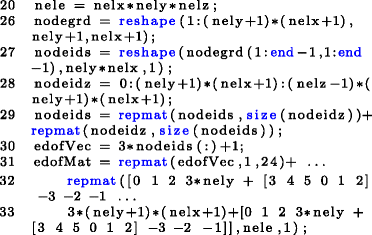where nele is the total number of elements, nodegrd contains the node ID of the first grid of nodes in the x-y plane (for z = 0), the column vector edofVec contains the node IDs of the first node at each element, and the connectivity matrix edofMat of size nele × 24 containing the node IDs for each element. For the volume in Fig. 2, nelx=4, nely=1, and nelz=2, which results in

$$\texttt{edofMat} = \left[\begin{array}{lllllll} 4 & 5 & 6 & \cdots & 31 & 32 & 33 \\ 10 & 11 & 12 & \cdots & 37 & 38 & 39\\ 16 & 17 & 18 & \cdots & 43 & 44 & 45\\ 22 & 23 & 24 & \cdots & 49 & 50 & 51\\ 34 & 35 & 36 & \cdots & 61 & 62 &63\\ 40 & 41 & 42 & \cdots & 67 & 68 & 69\\ 46 & 47 & 48 & \cdots & 73 & 74 & 75\\ 52 & 53 & 54 & \cdots & 79 & 80 & 81\\ \end{array}\right] \begin{array}{cl} \leftarrow \text{Element } 1& \\ \leftarrow \text{Element } 2& \\ \leftarrow \text{Element } 3& \\ \leftarrow \text{Element } 4& \\ \leftarrow \text{Element } 5& \\ \leftarrow \text{Element } 6& \\ \leftarrow \text{Element } 7& \\ \leftarrow \text{Element } 8&. \\ \end{array}$$

The element connectivity matrix edofMat is used to assemble the global stiffness matrix K as follows: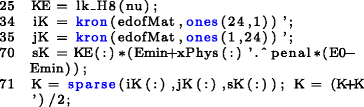The element stiffness matrix KE (size 24 × 24) is obtained from the lk_H8 subroutine (lines 99-146). Matrices iK (size 24 nele × 24) and jK (size nele × 242), reshaped as column vectors, contain the rows and columns identifying the 24 × 24 × nele DOFs in the structure. The three-dimensional array xPhys (size nely × nelx × nelz) corresponds to the physical densities. The matrix sK (size 242 × nele) contains all element stiffness matrices. The assembly procedure of the (sparse symmetric) global stiffness matrix K (line 71) avoids the use of nested for loops.

Finally, the nodal displacement vector U is obtained from the solution of the equilibrium equation (16) by pre-multiplying the inverse of the stiffness matrix K and the vector of nodal forces F,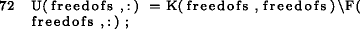where the indices freedofs indicate the unconstrained DOFs. For the cantilevered structure in Fig. 2, the constrained DOFs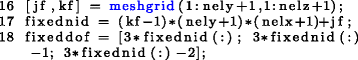where jf, and kf are the coordinate of the fixed nodes, fixednid are the node IDs, and fixeddof are the location of the DOFs. The free DOFs, are then defined as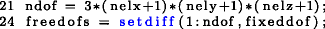where ndof is the total number of DOFs. By default, the code constraints the left face of the prismatic structure and assigns a vertical load to the structure’s free-lower edge as depicted in Fig. 2. The user can define different load and support DOFs by changing the corresponding node coordinates (lines 12 and 16). Several examples are presented in Section 6.

## Optimization problem formulation

Three representative topology optimization problems are described in this section, namely: minimum compliance, compliant mechanism synthesis, and heat conduction.

### Minimum compliance

The objective of the minimum compliance problem is to find the material density distribution $$\tilde {\mathrm {x}}$$ that minimizes the structure’s deformation under the prescribed support and loading condition. The structure’s compliance, which provides a global measure of deformation, is defined as

$$c(\tilde{\mathbf{x}}) = {\mathbf{F}}^{\text{{T}}}\mathbf{U}(\tilde{\mathbf{x}}),$$
(17)

where F is the vector of nodal forces and U () is the vector of nodal displacements. Incorporating a volume constraint, the minimum compliance optimization problem is

$$\begin{array}{cccc} \text{find} &&& {\mathbf{x}} = {[x_{1},x_{2},\dotsc,x_{e},\dotsc,x_{n}]}^{\text{{T}}} \\ \text{minimize} &&& c(\tilde{\mathbf{x}}) = {\mathbf{F}}^{\text{{T}}}\mathbf{U}(\tilde{\mathbf{x}}) \\ \text{subject to} &&& v(\tilde{\mathbf{x}}) = {\tilde{\mathbf{x}}}^{{\text{T}}}\mathbf{v} - \bar v \leqslant 0 \\ &&& \mathbf x \in \mathcal X,\quad \mathcal X = \{ \mathbf x \in \mathbb{R}^{n}: \mathbf{0} \leqslant \mathbf{x} \leqslant \mathbf{1}\},\\ \end{array}$$
(18)

where the physical densities = () are defined by (3), n is the number of elements used to discretize the design domain, v = [v 1,…,v n ]T is a vector of element volume, and $$\bar {v}$$ is the prescribed volume limit of the design domain. The nodal force vector F is independent of the design variables and the nodal displacement vector U() is the solution of K()U() = F.

The derivative of the volume constraint v() in (18) with respect to the design variable x e is given

$$\begin{array}{@{}rcl@{}} \dfrac {\partial v(\tilde{\mathbf{x}})}{\partial x_{e}} = {\sum}_{i \in N_{e}} \dfrac {\partial v(\tilde{\mathbf{x}})}{\partial \tilde x_{i}} \dfrac {\partial \tilde x_{i}}{\partial x_{e}} \end{array}$$
(19)

where

$$\begin{array}{@{}rcl@{}} \dfrac {\partial v(\tilde{\mathbf{x}})}{\partial \tilde{\mathrm{x}}_{i}} & = v_{i} \end{array}$$
(20)

and

$$\dfrac {\partial \tilde x_{i}}{\partial x_{e}} = \dfrac {H_{ie}v_{e}}{\sum_{j \in N_{i}} H_{ij}v_{j}}.$$
(21)

The code uses a mesh with equally sized cubic elements of unit volume, then v i =v j =v e =1.

The derivative of the compliance is

$$\begin{array}{@{}rcl@{}} \dfrac {\partial c(\tilde{\mathbf{x}})}{\partial x_{e}} = {\sum}_{i \in N_{e}} \dfrac {\partial c(\tilde{\mathbf{x}})}{\partial \tilde x_{i}} \dfrac {\partial \tilde x_{i}}{\partial x_{e}} \end{array}$$
(22)

where $$\partial \tilde {x_{i}} / \partial x_{e}$$ is given by (21) and

$$\begin{array}{@{}rcl@{}} \frac{\partial c(\tilde{\mathbf{x}})}{\partial \tilde{\mathrm{x}}_{i}} = {\mathbf{F}}^{\text{{T}}} \frac{\partial \mathbf{U}(\tilde{\mathbf{x}})}{\partial \tilde{\mathrm{x}}_{i}} \\ = {\mathbf{U}(\tilde{\mathbf{x}})}^{\text{{T}}} \mathbf{K}(\tilde{\mathbf{x}}) \frac{\partial \mathbf{U}(\tilde{\mathbf{x}})}{\partial \tilde{\mathrm{x}}_{i}}. \end{array}$$
(23)

The derivative of (16) with respect to $$\tilde {\mathrm {x}}_{i}$$ is

$$\frac{\partial \mathbf{K}(\tilde{\mathbf{x}})}{\partial \tilde{\mathrm{x}}_{i}} \mathbf{U}(\tilde{\mathbf{x}}) + \mathbf{K}(\tilde{\mathbf{x}}) \frac{\partial \mathbf{U}(\tilde{\mathbf{x}})}{\partial \tilde{\mathrm{x}}_{i}} = \mathbf{0},$$
(24)

which yields

$$\frac{\partial \mathbf U(\tilde{\mathbf{x}})}{\partial \tilde{\mathrm{x}}_{i}} = -\mathbf{K}(\tilde{\mathbf{x}})^{-1} \frac{\partial \mathbf{K}(\tilde{\mathbf{x}})}{\partial \tilde{\mathrm{x}}_{i}} \mathbf U(\tilde{\mathbf{x}}).$$
(25)

Using (15),

$$\begin{array}{@{}rcl@{}} \frac{\partial \mathbf{K}(\tilde{\mathbf{x}})}{\partial \tilde{\mathrm{x}}_{i}} & = \frac{\partial}{\partial \tilde{\mathrm{x}}_{i}} {\sum}_{i=1}^{n} \left [ {\text E}_{{\text {min}}} + \tilde{\mathrm{x}}_{i}^{p} \left (\text E_{0} - \text E_{\text {min}} \right ) \right] \mathbf{K}^{0}_{i} \\ & = p \tilde{\mathrm{x}}_{i}^{p-1}\left (\text E_{0} - \text E_{\min} \right ) \mathbf{K}^{0}_{i}. \end{array}$$
(26)

Using (25) and (26), (23) results in

$$\frac{\partial c(\tilde{\mathbf{x}})}{\partial \tilde{\mathrm{x}}_{i}} = - {\mathbf{U}(\tilde{\mathbf{x}})}^{\text{\scriptsize{T}}} \left [ p \tilde{\mathrm{x}}_{i}^{p-1}(\text E_{0} - \text E_{\min} )\mathbf{K}^{0}_{i} \right ] \mathbf U(\tilde{\mathbf{x}}).$$
(27)

Since $$\mathbf {k}^{0}_{i}$$ is the global version of an element matrix, (27) may be transformed from the global level to the element level, obtaining

$$\frac{\partial c(\tilde{\mathbf{x}})}{\partial \tilde{\mathrm{x}}_{i}} = - {\mathbf{u}_{i}(\tilde{\mathbf{x}})}^{{\text{T}}} \left [ p \tilde{\mathrm{x}}_{i}^{p-1}(\text E_{0} - \text E_{\min} )\mathbf{k}^{0}_{i} \right ] \mathbf{u}_{i}(\tilde{\mathbf{x}}).$$
(28)

where u i is the element vector of nodal displacements. Since $$\mathbf {k}^{0}_{i}$$ is positive definite, ∂c()/∂x͂ i < 0.

The numerical implementation of minimum compliance problem can be done as the following: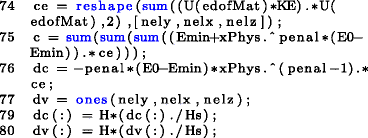The objective function in (18) is calculated in Line 75. The sensitivities of the objective function and volume fraction constraint with respect to the physical density are given be lines 76-77. Finally, the chain rule as stated in (22) is deployed in lines 79-80.

### Compliant mechanism synthesis

A compliant mechanism is a morphing structure that undergoes elastic deformation to transform force, displacement, or energy (Bruns and Tortorelli 2001). A typical goal for a compliant mechanism design is to maximize the output port displacement. The optimization problem is

$$\begin{array}{cccc} \text{find} &&& \tilde{\mathbf{x}} = {[x_{1},x_{2},\dotsc,x_{e},\dotsc,x_{n}]}^{\text{\scriptsize{T}}} \\ \text{minimize} &&& c(\tilde{\mathbf{x}}) = -u_{\text{out}}(\tilde{\mathbf{x}}) = -{\mathbf{L}}^{\text{T}}\mathbf{U}({\tilde{\mathbf{x}}}) \\ \text{subject to} &&& v\left(\tilde{\mathbf{x}}\right)=\tilde{\mathbf{x}}^{\text{T}}\mathbf{v}-\bar{v}\leqslant0 \\ &&& \mathbf x \in \mathcal X,\quad \mathcal X = \{ \mathbf x \in \mathbb{R}^{n}: \mathbf{0} \leqslant \mathbf{x} \leqslant \mathbf{1}\},\\ \end{array}$$
(29)

where L is a unit length vector with zeros at all degrees of freedom except at the output point where it is one, and U () = K () −1 F.

To obtain the sensitivity of the new cost function c() in (29), let us define a global adjoint vector U d() from the solution of the adjoint problem

$$\mathbf{K}(\tilde{\mathbf{x}}) \mathbf U_{d}(\tilde{\mathbf{x}}) = - \mathbf{L}.$$
(30)

Using (30) in (29), the objective function can be expressed as

$$c(\tilde{\mathbf{x}}) = {\mathbf U_{d}(\tilde{\mathbf{x}})}^{\text{T}} \mathbf{K}(\tilde{\mathbf{x}}) \mathbf{U}(\tilde{\mathbf{x}}).$$
(31)

The derivative of c() with respect to the design variable x e is again obtained by the chain rule,

$$\dfrac {\partial c(\tilde{\mathbf{x}})}{\partial x_{e}} = {\sum}_{i \in N_{e}} \dfrac {\partial c(\tilde{\mathbf{x}})}{\partial \tilde x_{i}} \dfrac {\partial \tilde x_{i}}{\partial x_{e}},$$

where $${\partial \tilde x_{i}}/{\partial x_{e}}$$ is described by (21), and ∂c()/∂x͂ i can be obtained using direct differentiation. The use of the interpolation given by (6) yields an expression similar to the one obtained in (28),

$$\frac {\partial c(\tilde{\mathbf{x}})} {\partial \tilde x_{i}} = \mathbf u_{di}(\tilde{\mathbf{x}})^{\text{T}} \left [ p \tilde x_{i}^{p-1}(\text E_{0} - \text E_{\min}) \mathbf{k}^{0}_{i} \right ] \mathbf u_{i}(\tilde{\mathbf{x}}).$$
(32)

where $$\mathbf u_{di}({\mathbf {k}}_{i}^{0})$$ is the part of the adjoint vector associated with element i. In this case, $${\partial c({\mathbf {k}}_{i}^{0})}/{\partial \tilde {\mathrm {x}}_{i}}$$ may be positive or negative.

The numerical implementation of the objective function (31) and sensitivity (32) are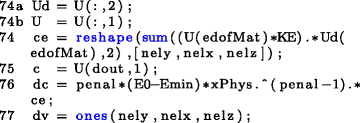Vector Ud (Line 74a) is the dummy load displacement field and vector U (line 74b) is the input load displacement. The codes for the implementation of chain rule are not shown above since they are same as lines 79-80.

### Heat conduction

Heat in physics is defined as energy transferred between a system and its surrounding. The direct microscopic exchange of kinetic energy of particles through the boundary between two systems is called diffusion or heat conduction. When a body is at a different temperature from its surrounding, heat flows so that the body and the surroundings reach the same temperature. This condition is known as thermal equilibrium. The equilibrium condition for heat transfer in finite element formulation is described by

$$\mathbf K({\mathbf{k}}_{i}^{0}) \mathbf U({\mathbf{k}}_{i}^{0}) = \mathbf F,$$

where $$\mathbf U(\mathbf {k}_{i}^{0})$$ now donates the finite element global nodal temperature vector, F donates the global thermal load vector, and $$\mathbf K({\mathbf {k}}_{i}^{0})$$ donates the global thermal conductivity matrix. For a material with isotropic properties, conductivity is the same in all directions.

The optimization problem for heat conduction is

$$\begin{array}{cccc} \text{find} &&& {\mathbf{k}}_{i}^{0} = {[x_{1},x_{2},\dotsc,x_{e},\dotsc,x_{n}]}^{\text{\scriptsize{T}}} \\ \text{minimize} &&& c({\mathbf{k}}_{i}^{0}) = \mathbf F^{\text T} \mathbf U({\mathbf{k}}_{i}^{0}) \\ \text{subject to} &&&v\left(\tilde{\mathbf{x}}\right)=\tilde{\mathbf{x}}^{\text{T}}\mathbf{v}-\bar{v}\leqslant0 \\ &&& \mathbf x \in \mathcal X,\quad \mathcal X = \{ \mathbf x \in \mathbb{R}^{n}: \mathbf{0} \leqslant \mathbf{x} \leqslant \mathbf{1}\},\\ \end{array}$$
(33)

where U() = K ()−1 F, and K() is obtained by the assembly of element thermal conductivity matrices $$\mathbf {k}_{i}(\tilde {\mathrm {x}}_{i})$$. Following the interpolation function in (6), the element conductivity matrix is expressed as

$$\mathbf k_{i}(\tilde{\mathrm{x}}_{i}) = \left[ k_{\min} + (k_{0}- k_{\min}) \tilde{\mathrm{x}}_{i}^{p}\right] \mathbf{k}_{i}^{0},$$
(34)

where k min and k 0 represent the limits of the material’s thermal conductivity coefficient and $$\mathbf {k}_{i}^{0}$$ donates the element conductivity matrix. Note that (34) may be considered as the distribution of two material phases: a good thermal conduction (k 0) and the other a poor conductor (k min).

The sensitivity analysis of the cost function in (33) is given by

$$\begin{array}{@{}rcl@{}} \dfrac {\partial c(\tilde{\mathbf{x}})}{\partial x_{e}} = {\sum}_{i \in N_{e}} \dfrac {\partial c(\tilde{\mathbf{x}})}{\partial \tilde x_{i}} \dfrac {\partial \tilde x_{i}}{\partial x_{e}}, \end{array}$$

where $${\partial \tilde x_{i}}/{\partial x_{e}}$$ is described by (21) and

$$\frac {\partial c(\tilde{\mathbf{x}})} {\partial \tilde x_{i}} = - \mathbf u_{i}(\tilde{\mathbf{x}})^{\text{T}} \left [(k_{0} - k_{\min}) p \tilde x_{i}^{p-1} \mathbf{k}_{i}^{0}\right ] \mathbf u_{i}(\tilde{\mathbf{x}}).$$
(35)

The numerical implementation only requires an optional change in the material property name: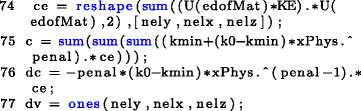where k0 and kmin are the limits of the material’s thermal conductivity. The chain rule is applied same as before.

## Optimization algorithms

Non-linear programming (NLP) problems, such as minimum compliance (18), compliant mechanism (29), and heat conduction (33), can be addressed using sequential convex approximations such as sequential quadratic programming (SQP) (Wilson 1963) and the method of moving asymptotes (MMA) (Svanberg 1987). The premise of these methods is that, given a current design x (k), the NLP algorithm is able to find a convex approximation of the original NLP problem from which an improved design x (k+1) can be derived. The nature of the problem’s approximation is determined by the type of algorithm, e.g., quadratic programming (QP) or MMA. An special case of the latter approach, which is historically older than SQP and MMA, is the optimality criterion (OC) method. This method still find its place in topology optimization due to its numerical simplicity and numerical efficiency (Christensen and Klarbring 2009). The following sections presents the implementation of the SQP, MMA, and OC methods to the solution of the minimum compliance topology optimization problems presented in this paper.

### Sequential quadratic programming

A QP problem has a quadratic objective function and linear constraints (Nocedal and Wright 2006). Given the current design x (k) and all corresponding active constraints, the QP approximation of the minimum compliance problem in (18) can be expressed as

$$\begin{array}{cccc} \text{find} & & & \mathbf{d} \\ \text{minimize} & & & \frac{1}{2} {\mathbf{d}}^{\text{{T}}}\nabla^{2}c^{(k)}\mathbf{d} + {\nabla c^{(k)}}^{\text{T}}\mathbf{d} \\ \text{subject to} & & & \mathbf{Ad} = \mathbf{0}, \end{array}$$
(36)

where c (k) is the value of the objective function evaluated at x (k) and d = xx (k), and A is the matrix of active constraints. The optimality and feasibility conditions of (36) yield

\begin{aligned} \nabla^{2}c^{(k)} \mathbf{d} + {\mathbf{A}}^{\text{{T}}} \boldsymbol{\lambda} && = &&& - \nabla c^{(k)} \\ \mathbf{Ad} && = &&&\mathbf{0} \end{aligned}
(37)

This SQP approach, referred to as the active set algorithm (Nocedal and Wright 2006), allows to determine a step $$\tilde {d}^{(k)}$$ from the solution of the of system of linear equations in (37) expressed as

$$\left[ \begin{array}{cc} \nabla^{2}c^{(k)} & {\mathbf{A}}^{\text{\scriptsize{T}}} \\ \mathbf{A} & \mathbf{0} \end{array} \right] \left[ \begin{array}{c} \mathbf{d}^{(k)} \\ \boldsymbol{\lambda}^{(k)} \end{array} \right] = \left[ \begin{array}{c} - \nabla c^{(k)} \\ \mathbf{0} \end{array} \right].$$
(38)

The updated design is given by

$$\mathbf{x}^{\left(k+1\right)}=\mathbf{x}^{\left(k\right)}+\alpha^{\left(k\right)}\mathbf{d}^{\left(k\right)},$$
(39)

where the step size parameter α (k) is determined by a line search procedure. The Hessian ∇2 c (k) can be numerically approximated but, for the problems considered in this paper, one can determined the closed-form expression (see Appendix B), which is given by

\frac{\partial^{2}c}{\partial \tilde{\mathrm{x}}_{i}\partial\tilde{\mathrm{x}}_{j}}= \left\{\begin{array}{llll} 0, & i\neq j,\\ \!\begin{aligned} & 2\left[p\tilde{\mathrm{x}}_{i}^{p-1}(\text{E}_{0}-\text{E}_{\min})\right]^{2} \\ & \left[\text{E}_{\min}+\tilde{\mathrm{x}}_{i}^{p}(\text{E}_{0}-\text{E}_{\min})\right]^{-1}\vec u_{i}^{\text{T}}\mathbf{k_{i}^{0}}\mathbf u_{i}, \end{aligned} & i=j.\\ \end{array}\right.
(40)

This line-search, active-set SQP algorithm is summarized in Algorithm 1.

Algorithm 1 SQP Algorithm
Choose an initial feasible design x (0); set k←0;
while (convergence criteria are not met) do
Evaluate c (k), ∇c (k)(28), ∇2 c (k)(40);
Identify active constraint matrix A in (36);
Solve for d (k)(38);
Find appropriate step size α (k);
Set x (k+1)x (k) + α (k) d (k);
Set kk+1
end while

The implementation of (40) in the program can be done in just two lines, since the term $$\mathbf {u}_{i}^{\text {T}}+\mathbf {k}_i^0 +\mathbf u_{i}$$ has already been calculated, namely matrix ce in line 74: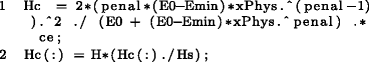Finally, Matlab has built-in constrained NLP solver fmincon. The implementation of using fmincon as an optimizer in our top3d program is quite easy, but need some reconstructions of the program (one needs to divide program into different subfunctions, e.g., objective function, constraint function, Hessian function). To further assist on the implementation of an SQP strategy, the reader can find a step-by-step tutorial on our website http://top3dapp.com.

### Method of moving asymptotes

The MMA algorithm (Svanberg 1987) was proposed to adjust the curvature of the convex linearization (CONLIN) method introduced by Fleury (1989). Give the current design x (k) the MMA approximation of the minimum compliance problem in (18) yields to the following linear programming problem:

$$\begin{array}{cccc} \text{find} & & & \mathbf{x}\\ \text{minimize} & & & -{\sum}_{i=1}^{n}\left[\frac{\left(x_{i}^{\left(k\right)}-L_{i}^{\left(k\right)}\right)^{2}}{x_{i}-L_{i}^{\left(k\right)}}\frac{\partial c}{\partial x_{i}}\left(\tilde{\mathbf{x}}^{\left(k\right)}\right)\right]\\ \text{subject to} & & & {\tilde{\mathbf{x}}}^{\text{T}}\mathbf{v}-\bar{v}\leqslant0 \\ & & & \mathbf{x}\in\mathcal{X}^{(k)}, \end{array}$$
(41)

where

$$\begin{array}{@{}rcl@{}} \mathcal{X}^{(k)} = \left\{ \mathbf{x}\in\mathcal{X}\,\vert\,0.9L_{i}^{(k)} + 0.1x_{i}^{(k)}\leqslant x_{i}\leqslant0.9U_{i}^{(k)}\right. \\ \left.+ 0.1x_{i}^{\text{(k)}},\, i=1,\ldots,n \right\}. \end{array}$$
(42)

The lower and upper asymptotes $$L_{i}^{\left (k\right )}$$ and $$U_{i}^{\left (k\right )}$$ are iteratively updated to mitigate oscillation or improve convergence rate. The heuristic rule proposed by Svanberg (1987) is as follows: For k = 1 and k = 2,

\begin{aligned} U_{i}^{\left(k\right)}+L_{i}^{\left(k\right)} & = 2x_{i}^{\left(k\right)},\\ U_{i}^{\left(k\right)}-L_{i}^{\left(k\right)} & = 1. \end{aligned}
(43)

For $$k\geqslant 3$$,

\begin{aligned} U_{i}^{\left(k\right)}+L_{i}^{\left(k\right)} & = 2x_{i}^{\left(k\right)}, \\ U_{i}^{\left(k\right)}-L_{i}^{\left(k\right)} & = \gamma_{i}^{\left(k\right)}, \end{aligned}
(44)

where

\begin{aligned} &\gamma_{i}^{\left(k\right)}=\\ &\left\{\begin{array}{llllll} 0.7 & \left(x_{i}^{\mathrm{\ensuremath{\left(k\right)}}}-x_{i}^{\mathrm{\ensuremath{\left(k-1\right)}}}\right)\left(x_{i}^{\mathrm{\ensuremath{\left(k-1\right)}}}-x_{i}^{\mathrm{\ensuremath{\left(k-2\right)}}}\right)<0\\ 1.2 & \left(x_{i}^{\mathrm{\ensuremath{\left(k\right)}}}-x_{i}^{\mathrm{\ensuremath{\left(k-1\right)}}}\right)\left(x_{i}^{\mathrm{\ensuremath{\left(k-1\right)}}}-x_{i}^{\mathrm{\ensuremath{\left(k-2\right)}}}\right)>0\\ \ 1 & \left(x_{i}^{\mathrm{\ensuremath{\left(k\right)}}}-x_{i}^{\mathrm{\ensuremath{\left(k-1\right)}}}\right)\left(x_{i}^{\mathrm{\ensuremath{\left(k-1\right)}}}-x_{i}^{\mathrm{\ensuremath{\left(k-2\right)}}}\right)=0 \end{array}\right. \end{aligned}
(45)

Note from (45) that the signs of three successive iterations are stored. If the signs are opposite, meaning x i oscillates, the two asymptotes are brought closer to $$x_{i}^{\left (k\right )}$$ to have a more conservative MMA approximation. On the other hand, if the signs are same, the two asymptotes are extended away from $$x_{i}^{\left (k\right )}$$ in order to speed up the convergence. The MMA algorithm is explained in Algorithm 2.

Algorithm 2 MMA Algorithm
Choose an initial feasible design x (0); set k←0;
while (convergence criteria are not met) do
if k = 1 or k = 2 then
Update $${L_{i}^{k}}$$, and $${U_{i}^{k}}$$ using (43);
else
Update $${L_{i}^{k}}$$, and $${U_{i}^{k}}$$ using (44) and (45);
Find appropriate step size α (k);
end if
Calculate derivate (28);
Solve the MMA subproblem (41) to obtain $$\tilde {\mathrm {x}}^{(k+1)}$$;
Set x (k−2)x (k−1), x (k−1)x (k), x (k)x (k+1);
Set kk+1;
end while

The MMA algorithm is available for Matlab (mmasub). The reader may obtain a copy by contacting Prof. Krister Svanberg (http://www.math.kth.se/%7Ekrille/Welcome.html) from KTH in Stockholm Sweden. Although mmasub has total of 29 input and output variables, its implementation for top3d is straightforward. The details can be found at http://top3dapp.com.

### Optimality criteria

A classical approach to structural optimization problems is the Optimality Criteria (OC) method. The OC method is historically older than sequential approximation methods such as Sequential Linear Programming (SLP) or SQP. The OC method is formulated on the grounds that if constraint 0x1 is inactive, then convergence is achieved when the KKT condition

$$x_{i}^{\left (k\right )}$$
(46)

is satisfied for k = 1,…, n, where λ is the Lagrange multiplier associated with the constraint v(). This optimality condition can be expressed as B e =1, where

$$B_{e} = - \frac{\partial c(\tilde{\mathbf{x}})}{\partial x_{e}} \left(\lambda \frac{\partial v(\tilde{\mathbf{x}})}{\partial x_{e}} \right)^{-1}.$$
(47)

The code implements the OC updating scheme proposed by (Bendsøe 1995) to update design variables:

$$x_{e}^{\text {new}} = \left\{\begin{array}{lllll} \max(0,~x_{e} - m), & \mathrm{if } x_{e} B_{e}^{\eta} \leqslant \max(0,~x_{e} - m), \\ \min(1,~x_{e} + m), & \mathrm{if } x_{e} B_{e}^{\eta} \geqslant \min(1,~x_{e} - m), \\ x_{e} B_{e}^{\eta}, & \text{otherwise}, \end{array}\right.$$
(48)

where m is a positive move-limit, and η is a numerical damping coefficient. The choice of m = 0.2 and η = 0.5 is recommended for minimum compliance problems (Bendsøe 1995; Sigmund 2001). For compliant mechanisms, η = 0.3 improves the convergence of the algorithm. The only unknown in (48) is the value of the Lagrange multiplier λ, which satisfies that

$$v(\tilde{\mathbf{x}}({\mathbf{x}}^{\text {new}}(\lambda))) = 0.$$
(49)

Numerically, λ is found by a root-finding algorithm such as the bisection method. Finally, the termination criteria are satisfied when a maximum number of iterations is reached or

$$|| {\mathbf{x}}^{\text {new}} - {\mathbf{x}} ||_{\infty} \leqslant \epsilon,$$
(50)

where the tolerance 𝜖 is a relatively small value, for example 𝜖 = 0.01.

The numerical implementation begins with the initialization of design and physical variables,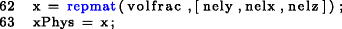where volfrac represents the volume fraction limit. Initially, the physical densities are assigned a constant uniform value, which is iteratively updated following the OC updating scheme (Algorithm 3).

Algorithm 3 OC Algorithm
Choose an initial design x (k); set k←0;
while (convergence criteria are not met) do
FE-analysis using (16) to obtain the corresponding
nodal displacement U (k);
Compute objective function, e.g., compliance c, out-
put displacement u out;
Sensitivity analysis by using the equations as dis-
cussed in Section 4;
Apply filter techniques, e.g. (3) in Section 2.3 or any
other filters like those discussed in Section 6.1.4
Update design variables using (48) to obtain x (k+1);
Set x (k+1)x (k); Set kk+1;
end while

## Numerical examples

The code is executed Matlab with the following command: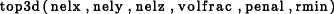where nelx, nely, and nelz are number of elements along x, y, and z directions, volfrac is the volume fraction limit ($$\bar v$$), penal is the penalization power (p), and rmin is filter size (R). User-defined variables are set between lines 3 and 18. These variables determine the material model, termination criteria, loads, and supports. The following examples demonstrate the application of the code to minimum compliance problems, and its extension to compliant mechanism synthesis and heat condition.

### Minimum compliance

By default, the code solves a minimum compliance problem for the cantilevered beam in Fig. 4. The prismatic design domain is fully constrained in one end and a unit distributed vertical load is applied downwards on the lower free edge. Figure 4 shows the topology optimization results for solving minimum compliance problem with the following Matlab input lines: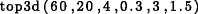Fig. 4

#### Boundary conditions

The boundary conditions and loading conditions are defined in lines 12-18. Since the node coordinates and node numbers are automatically mapped by the program, defining different boundary conditions is very simple. To solve a 3D wheel problem as shown in Fig. 5, which is constrained by planar joint on the corners with a downward point load in the center of the bottom, the following changes need to be made:Fig. 5

Firstly, changing loading conditions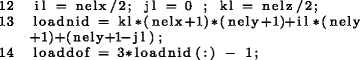Secondly, defining the corresponding boundary conditions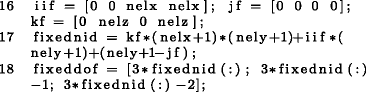then the problem can be promoted by line:#### Multiple load cases

In order to solve a multiple load cases problem, as shown in Fig. 6, a few changes need to be made. First, the loading conditions (line 12) are changed correspondingly: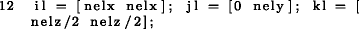Fig. 6

Also the force vector (line 22) and displacement vector (line 23) become more than one column: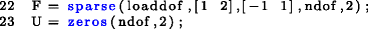The objective function is now the sum of different load cases

$$c(\tilde{\mathbf{x}}) = {\sum}_{l=1}^{M} c_{l}(\tilde{\mathbf{x}}) = {\sum}_{l=1}^{M} \mathbf{F}_{l}^{\text{T}}\mathbf{U}_{l}\left(\tilde{\mathbf{x}}\right)$$
(51)

where M is the number of load cases.

Then lines 74–76 are substituted with lines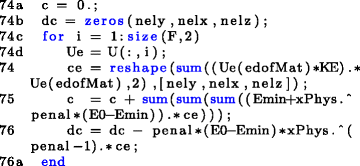This example is promoted by the line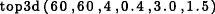#### Active and passive elements

In some designs, some elements may be desired to be solid or void. A nely × nelx × nelz matrix with ones at elements desired to be solid, and a nely × nelx × nelz matrix with zeros at elements desired to be void can be added to the program. To solve the problem as shown in Fig. 7, the passive elements need to be defined first by adding the following lines after line 62: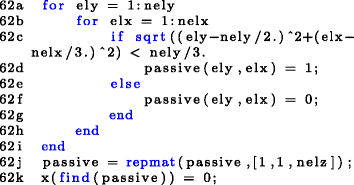Fig. 7

In addition, one line is added in the OC subroutine under line 85: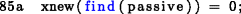The optimized beam shown in Fig. 7 is promoted by the line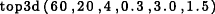#### Alternative filters

In the topology optimization, filters are introduced to avoid numerical instabilities. Different filtering techniques may result different discreteness of the final solutions, and sometimes may even contribute to different topologies. In addition to density filter, in the literatures there are bunch of different filtering schemes. For example, sensitivity filter (Sigmund 1994, 1997), morphology based black and white filters (Sigmund 2007), filtering technique using Matlab built-in function conv2 (Andreassen et al. 2011), filtering based on Helmholtz type differential equations (Andreassen et al. 2011), Heaviside filter (Guest et al. 2004, 2011), and gray scale filter (Groenwold and Etman 2009). All the filters pursue a simple goal to achieve black-and-white structures. Two of them are chosen, which stand for classic and better performance, as well as easy implementation.

### Sensitivity filter

Sigmund (1994, 1997) introduced the sensitivity filter. The working principle is to replace the real sensitivities by the filtered sensitivities. In addition, the sensitivity filter is implemented in the 99-line code as the default filtering scheme. It modifies the element sensitivity during every iteration by the following

$$\dfrac {\widehat {\partial c(\mathbf{x})}}{\partial x_{i}} = \dfrac {1}{\max (\gamma,x_{i}) {\sum}_{j \in N_{i}} H_{ij}} {\sum}_{j \in N_{i}} H_{ij} ~x_{j}~ \dfrac {\partial c(\mathbf{x})}{\partial x_{j}}.$$

where γ (=10−3) is a small number in order to avoid division by zero.

The implementation of the sensitivity filter can be achieved by adding and changing a few lines.

Change line 2 by adding one input variable ft (ft=1 for density filter, ft=2 for sensitivity filter)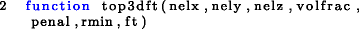Adding the sensitivity filter to the program, by changing lines 79-80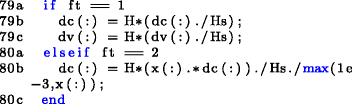Changing the design variable update strategy (line 86) in the optimal search procedure### Gray scale filter

A simple non-linear gray-scale filter or intermediate density filter has been proposed by Groenwold and Etman (2009) to further achieve black-and-white topologies. The implementation of the gray scale filter is by changing the OC update scheme as the following

$$x_{i}^{\text {new}} = \left\{\begin{array}{lllll} \max(0,~x_{i} - m), & \mathrm{if } x_{i} B_{i}^{\eta} \leqslant \max(0,~x_{i} - m) \\ \min(1,~x_{i} + m), & \mathrm{if } x_{i} B_{i}^{\eta} \geqslant \min(1,~x_{i} - m) \\ (x_{i} B_{i}^{\eta})^{q}, & \text{otherwise} \end{array}\right.$$
(52)

The standard OC updating method is a special case of (52) with q = 1. A typical value of q for the SIMP-based topology optimization is q = 2.

The implementation of the gray scale filter to the code can be done as follows:

Adding one input variable q to the program (line 2)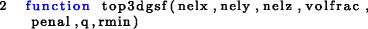Change the OC updating method (line 85) to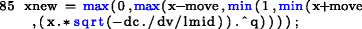The factor q should be increased gradually by adding one line after line 68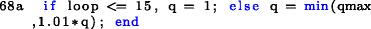Figure 8 demonstrates the optimized beams applying different filtering techniques. As can be seen from final results, both sensitivity filter, density filter and gray scale filter suppress checkerboard patterns. The gray scale filter combines with the sensitivity filter provides the most black-and-white solution.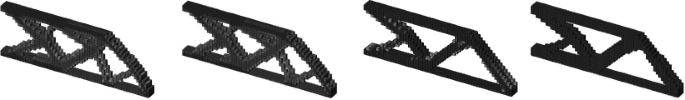Fig. 8

#### Iterative solver

If the finite element mesh size becomes large, the traditional direct solver (line 72) used to address the finite element analysis is suffered by longer solving time and some other issues. However, iterative solver (Hestenes and Stiefel 1952; Augarde et al. 2006) can solve large-scale problems efficiently. To this end, line 72 is replaced by a built-in Matlab function pcg, called preconditioned conjugate gradients method, as shown in the following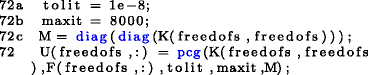Direct solver is a special case by setting the preconditioner (line 72c) to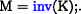Table 3 gives the comparison of two different finite element analysis solvers. As shown in the table, a speed up factor of 30.81 has been measured when solving large scale problem. Hence, the iterative solver is more suitable for large-scale problems, and vice versa.

Some examples include a cantilever beam, the Messerchimitt-Bölkow-Blohm (MBB) beam and L-shape problems (Table ??) are solved by using iterative solver and applying gray scale filter. The underlined triangle represents a three-dimensional planar joint.

#### Continuation strategy

Convexity is a very preferable property since every local minima is also the global minima, and what the program is solving for is the global minima. Unfortunately, the use of SIMP method to achieve binary solution will destroy the convexity of the optimization problem. For such problems, it is possible that for different starting points the program converges to totally different local minima. In order to penalize intermediate densities and mitigate the premature convergence to one of the multiple local minima when solving the non-convex problem, one could perform a continuation step. As previously presented by Groenwold and Etman (2010), the continuation step is given as

$$p^{k} = \left\{\begin{array}{llllll} 1 & k \leqslant 20,\\ {\min} \{ p^{\max},1.02p^{k-1} \} & k > 20, \end{array}\right.$$
(53)

where k is the iteration number, and p max is the maximum penalization power.

Though this methodology is not proven to converge to the global optimum, it regularizes the algorithm and allows the comparison of different optimization strategies.

Implementing the continuation strategy is done by adding a single line after line 68: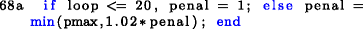### Compliant mechanism synthesis

A compliant mechanism problem involves loading cases: input loading case and dummy loading case. The code also needs to implement a new objective function and its corresponding sensitivity analysis. To demonstrate this implementation, let us consider a three-dimensional force inverter problem as shown in Fig. 9. With an input load defined in the positive direction, the design goal is to maximize the negative horizontal output displacement. Both the top face and the side force are imposed with symmetric constraints; i.e., nodes can only move within the plane.Fig. 9

The new loading conditions as well as input and output points are defined as follows: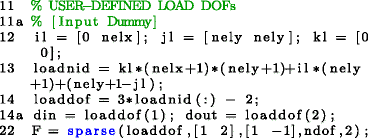and the boundary conditions are defined as below: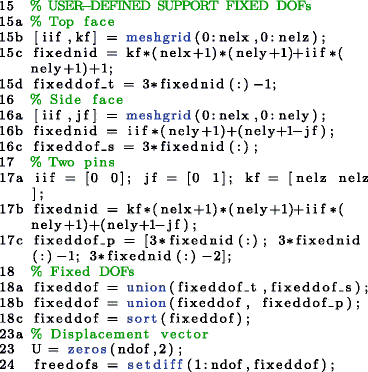The external springs with stiffness 0.1 are added at input and output points after line 71.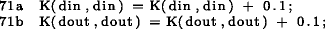The expressions of the objective function (31) and sensitivity (32) are modified in lines 74-76.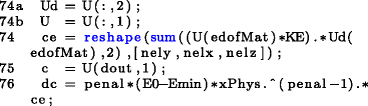The convergence criteria for the bi-sectioning algorithm (lines 82-83) is improved by the following lines: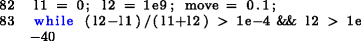To improve the convergence stability, the damping factor of OC-method changes from 0.5 to 0.3 and also takes the positive sensitivities into account, then line 85 is changed to: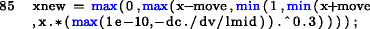The final design shown in Fig. 10 is promoted by the line in the Matlab: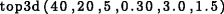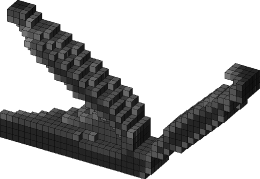Fig. 10

### Heat conduction

The implementation of heat conduction problems is not more complex than the one for compliant mechanism synthesis since the number of DOF per node is one rather than three. Following the implementation of heat conduction problems in two dimensions (Bendsøe and Sigmund 2003), the implementation for three dimension problems is suggested in the following steps.

First, the elastic material properties (lines 8-10) are changed to the thermal conductivities of materials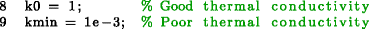Furthermore, the boundary conditions for the heat condition problem, i.e., a rectangular plate with a heat sink on the middle of top face and all nodes are given a thermal load as shown in Fig. 11, are changed corresponding (lines 10-18).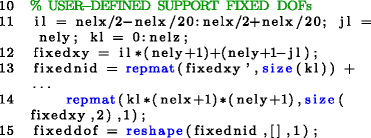Fig. 11

Also, since there is only one DOF per node in heat condition problems, some variables need to change correspondingly, such as ndof, edofMat.

Change the total number of DOFs and the force vector in lines 21–22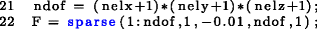The element conductivity matrix is called in line 25 by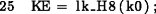and it is defined in lines 99–145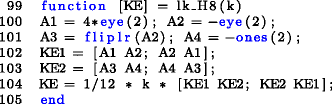The finite element connectivity matrix edofMat is changed in lines 30–35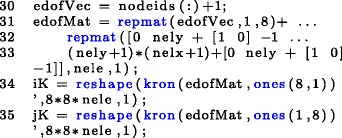The global conductivity matrix is assembled in a different way, hence line 70 need to change as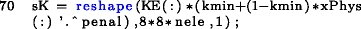The evulation of the objective function and analysis of the sensitivity are given in lines 75–76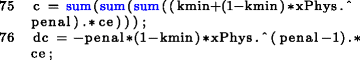The optimized topology is derived as shown in Fig. 12 by promoting the following line in the Matlab: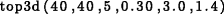Fig. 12

## Conclusions

This paper presents Matlab the analytical elements and the numerical implementation of an academic three-dimensional structural topology optimization algorithm referred to as top3d. In this topology optimization algorithm, the problem formulation follows a density-based approach with a modified SIMP interpolation for physical densities. The finite element formulation makes use of eight-node hexahedral elements for which a closed-form expression of the element stiffness matrix is derived and numerically implemented. The hexahedral finite elements are used to uniformly discretize a prismatic design domain and solve three related topology optimization problems: minimum compliance, compliant mechanism, and heat conduction problems. For each problem, this paper includes the analytical derivation of the sensitivity coefficients used by three gradient-based optimization algorithms: SQP, MMA, and OC, which is implemented by default. For the implementation of SQP, this paper derives an analytic expression for the second order derivative.

The use of top3d is demonstrated through several numerical examples. These examples include problems with a variety of boundary conditions, multiple load cases, active and passive elements, filters, and continuation strategies to mitigate convergence to a local minimum. The architecture of the code allows the user to map node coordinates of node degrees-of-freedom boundary conditions. In addition, the paper provides a strategy to handle large models with the use of an iterative solver. For large-scale finite-element models, the iterative solver is about 30 times faster than the traditional direct solver. While this implementation is limited to linear topology optimization problems with a linear constraint, it provides a clear perspective of the analytical and numerical effort involved in addressing three-dimensional structural topology optimization problems. Finally, additional academic resources such the use of MMA and SQP are available at http://top3dapp.com.

## References

1. Aage N, Nobel-Jørgensen M, Andreasen CS, Sigmund O (2013) Interactive topology optimization on hand-held devices. Struct Multidiscip Optim 47(1):1–6

2. Allaire G (2001) Shape optimization by the homogenization method. Springer, New York

3. Allaire G, Kohn R (1993) Optimal design for minimum weight and compliance in plane-stress using extremal microstructures. Eur J Mech 12(6):839–878

4. Allaire G, Pantz O (2006) Structural optimization with freefem++. Struct Multidiscip Optim 32(3):173–181

5. Allaire G, Belhachmi Z, Jouve F (1996) The homogenization method for topology and shape optimization. single and multiple loads case. Eur J Finite Elem 5:649–672

6. Allaire G, Jouve F, Toader AM (2004) Structural optimization using sensitivity analysis and a level-set method. J Comput Phys 194(1):363–393

7. Andreassen E, Clausen A, Schevenels M, Lazarov BS, Sigmund O (2011) Efficient topology optimization in matlab using 88 lines of code. Struct Multidiscip Optim 43(1):1–16

8. Augarde C, Ramage A, Staudacher J (2006) An element-based displacement preconditioner for linear elasticity problems. Comput Struct 84(31–32):2306–2315

9. Bendsøe MP (1989) Optimal shape design as a material distribution problem. Struct Multidiscip Optim 1(4):193–202

10. Bendsøe MP (1995) Optimization of structural topology shape and material. Springer, New York

11. Bendsøe MP, Kikuchi N (1988) Generating optimal topologies in structural design using a homogenization method. Comput Methods Appl Mech Eng 71(2):197–224

12. Bendsøe MP, Sigmund O (2003) Topology optimization: theory, method and applications. Springer

13. Bruns TE, Tortorelli DA (2001) Topology optimization of non-linear elastic structures and compliant mechanisms. Comput Methods Appl Mech Eng 190(26–27):3443–3459

14. Challis VJ (2010) A discrete level-set topology optimization code written in matlab. Struct Multidiscip Optim 41(3):453–464

15. Christensen PW, Klarbring A (2009) An introduction to structural optimization. Springer

16. Duysinx P (1997) Layout optimization: A mathematical programming approach, DCAMM report. Technical report, Danish Center of Applied Mathematics and Mechanics, Technical University of Denmark, DK-2800 Lyngby

17. Fleury C (1989) CONLIN: an efficient dual optimizer based on convex approximation concepts. Struct Optim 1(2):81–89

18. Groenwold AA, Etman LFP (2009) A simple heuristic for gray-scale suppression in optimality criterion-based topology optimization. Struct Multidiscip Optim 39(2):217–225

19. Groenwold AA, Etman LFP (2010) A quadratic approximation for structural topology optimization. Int J Numer Methods Eng 82(4):505–524

20. Guest JK, Prevost JH, Belytschko T (2004) Achieving minimum length scale in topology optimization using nodal design variables and projection functions. Int J Numer Methods Eng 61:238–254

21. Guest JK, Asadpoure A, Ha SH (2011) Elimiating beta-continuation from heaviside projection and density filter algorithms. Struct Multidiscip Optim 44:443–453

22. Haber R, Jog C (1996) A new approach to variable-topology shape design using a constraint on perimeter. Struct Optim 11(1):1–12

23. Hassani B, Hinton E (1998) Homogenization and structural topology optimization: theory, practice and software. Springer

24. Hestenes MR, Stiefel E (1952) Methods of conjugate gradients for solving linear systems. J Res Natl Bur Stand 49(6)

25. Hunter W (2009) Predominantly solid-void three-dimensional topology optimisation using open source software. Master’s thesis, University of Stellenbosch

26. Jog C (2002) Topology design of structures using a dual algorithm and a constraint on the perimeter. Int J Numer Methods Eng 54(7):1007–1019

27. Kohn R, Strang G (1986a) Optimal design and relaxation of variational problems (part I). Commun Pure Applied Math 39(1):113–137

28. Kohn R, Strang G (1986b) Optimal design and relaxation of variational problems (part II). Commun Pure Appl Math 39(2):139–182

29. Kohn R, Strang G (1986c) Optimal design and relaxation of variational problems (part III). Commun Pure Appl Math 39(3):353–377

30. Liu Z, Korvink JG, Huang I (2005) Structure topology optimization: fully coupled level set method via FEMLAB. Struct Multidiscipl Optim 6(29):407–417

31. Mlejnek H (1992) Some aspects of the genesis of structures. Struct Optim 5(1–2):64–69

32. Nocedal J, Wright S (2006) Numerical optimization, 2nd edn. Springer

33. Schmit LA (1960) Structural design by systematic synthesis. In: 2nd ASCE conference of electrical compounds. Pittsburgh, pp 139–149

34. Sigmund O (1994) Design of material structures using topology optimization, PhD thesis, Technical University of Denmark

35. Sigmund O (1997) On the design of compliant mechanisms using topology optimization. Mech Struct Mach 25(4):495–526

36. Sigmund O (2001) A 99 line topology optimization code written in matlab. Struct Multidiscip Optim 21(2):120–127

37. Sigmund O (2007) Morphology-based black and white filters for topology optimization. Struct Multidiscip Optim 33:401–424

38. Sigmund O, Peterson J (1998) Numerical instabilities in topology optimization: a survey on procedures dealing with checkerboards, mesh-dependencies and local minima. Struct Optim 16:68–75

39. Sokół T (2011) A 99 line code for discretized michell truss optimization written in mathematica. Struct Multidiscip Optim 43(2):181–190

40. Suresh K (2010) A 199-line matlab code for pareto-optimal tracing in topology optimization. Struct Multidiscip Optim 42:665–679

41. Svanberg K (1987) The method of moving asymptotes-a new method for structural optimzation. Int J Numer Methods Eng 24:359–373

42. Talischi C, Paulino G H, Pereira A, Menezes IFM (2012a) Polymesher: a general-purpose mesh generator for polygonal elements written in matlab. Struct Multidiscip Optim 45:309–328

43. Talischi C, Paulino G H, Pereira A, Menezes IFM (2012b) Polytop: a matlab implementation of a general topology optimization framework using unstructured polygonal finite element meshes. Struct Multidiscip Optim 45:329–357

44. Wang MY, Chen S, Xia Q (2004) Structural topology optimization with the level set method. http://www2.acae.cuhk.edu.hk/cmdl/download.htm

45. Wilson RB (1963) A simplicial method for convex programming, PhD thesis, Harvard University

46. Zhou M, Rozvany G (1991) The COC algorithm, part II: topological, geometrical and generalized shape optimization. Comp Meth Appl Mech Eng 89:309–336

47. Zhou S, Wang MY (2005) 3d structural topology optimization with the simp method. http://www2.acae.cuhk.edu.hk/cmdl/download.htm

Download references

## Author information

Authors

### Corresponding author

Correspondence to Kai Liu.

## Appendix A: Symbolic expression of k 0

### Appendix A: Symbolic expression of k0

This appendix presents the analytical results of the element stiffness matrix k 0 as discussed in Section 3. This symbolic expression of k 0 is also been used by the program subroutine lk_H8.

Recall that, for an eight-node hexahedral element, the strain-displacement matrix B is defined by

$$\mathbf B = \left[\begin{array}{llllllll} \frac{\partial n_{1}(\xi_{e})}{\partial \xi_{1}} & 0 & 0 & \cdots & \frac{\partial n_{q}(\xi_{e})}{\partial \xi_{1}} & 0 & 0 \\[0.5em] 0 & \frac{\partial n_{1}(\xi_{e})}{\partial \xi_{2}} & 0 & \cdots & 0 & \frac{\partial n_{q}(\xi_{e})}{\partial \xi_{2}} \\[0.5em] 0 & 0 & \frac{\partial n_{1}(\xi_{e})}{\partial \xi_{3}} & \cdots & 0 & 0 & \frac{\partial n_{q}(\xi_{e})}{\partial \xi_{3}} \\[0.5em] \frac{\partial n_{1}(\xi_{e})}{\partial \xi_{2}} & \frac{\partial n_{1}(\xi_{e})}{\partial \xi_{1}} & 0 & \cdots & \frac{\partial n_{q}(\xi_{e})}{\partial \xi_{2}} & \frac{\partial n_{q}(\xi_{e})}{\partial \xi_{1}} & 0\\[0.5em] 0 & \frac{\partial n_{1}(\xi_{e})}{\partial \xi_{3}} & \frac{\partial n_{1}(\xi_{e})}{\partial \xi_{2}} & \cdots & 0 & \frac{\partial n_{q}(\xi_{e})}{\partial \xi_{3}} & \frac{\partial n_{q}(\xi_{e})}{\partial \xi_{2}} \\[0.5em] \frac{\partial n_{1}(\xi_{e})}{\partial \xi_{3}} & 0 & \frac{\partial n_{1}(\xi_{e})}{\partial \xi_{1}} & \cdots & \frac{\partial n_{q}(\xi_{e})}{\partial \xi_{3}} & 0 & \frac{\partial n_{q}(\xi_{e})}{\partial \xi_{1}}\\ \end{array}\right],$$

for e = 1,…,3 and q = 1,…,8. The corresponding shape functions n q in a natural coordinate systems ξ e are defined by

n_{q}(\xi_{e}) = \frac 1 8 \left \{ \begin{aligned} (1 - \xi_{1})(1 - \xi_{2})(1 - \xi_{3}) \\ (1 + \xi_{1})(1 - \xi_{2})(1 - \xi_{3}) \\ (1 + \xi_{1})(1 + \xi_{2})(1 - \xi_{3}) \\ (1-\xi_{1})(1+\xi_{2})(1-\xi_{3}) \\ (1-\xi_{1})(1-\xi_{2})(1+\xi_{3}) \\ (1+\xi_{1})(1-\xi_{2})(1+\xi_{3}) \\ (1+\xi_{1})(1+\xi_{2})(1+\xi_{3}) \\ (1-\xi_{1})(1+\xi_{2})(1+\xi_{3}) \\ \end{aligned} \right \}.

Substituting values to (11), the 24 × 24 element stiffness matrix $$\mathrm {k}_{i}^{0}$$ for an eight-node hexahedral element can be expressed as

$$\mathbf{k}_{i}^{0} = \frac{1}{(\nu + 1)(1-2\nu)} \left[\begin{array}{lllllll} \mathbf{k}_{1} && \mathbf{k}_{2} && \mathbf{k}_{3} && \mathbf{k}_{4} \\ {\mathbf{k}}^{\text{T}}_{2} && \mathbf{k}_{5} && \mathbf{k}_{6} && {\mathbf{k}}^{\text{T}}_{4} \\ {\mathbf{k}}^{\text{T}}_{3} && \mathbf{k}_{6} && {\mathbf{k}}^{\text{T}}_{5} && {\mathbf{k}}^{\text{T}}_{2} \\ \mathbf{k}_{4} && \mathbf{k}_{3} && \mathbf{k}_{2} && {\mathbf{k}}^{\text{{T}}}_{1} \end{array}\right],$$

where

$$\mathbf{k}_{1} = \left[\begin{array}{lllllllllllllll} k_{1} && k_{2} && k_{2} && k_{3} && k_{5} && k_{5} \\ k_{2} && k_{1} && k_{2} && k_{4} && k_{6} && k_{7} \\ k_{2} && k_{2} && k_{1} && k_{4} && k_{7} && k_{6} \\ k_{3} && k_{4} && k_{4} && k_{1} && k_{8} && k_{8} \\ k_{5} && k_{6} && k_{7} && k_{8} && k_{1} && k_{2} \\ k_{5} && k_{7} && k_{6} && k_{8} && k_{2} && k_{1} \\ \end{array}\right],$$
$$\mathbf{k}_{2} = \left[\begin{array}{llllllllllllll} k_{9} && k_{8} && k_{12} && k_{6} && k_{4} && k_{7} \\ k_{8} && k_{9} && k_{12} && k_{5} && k_{3} && k_{5} \\ k_{10} && k_{10} && k_{13} && k_{7} && k_{4} && k_{6} \\ k_{6} && k_{5} && k_{11} && k_{9} && k_{2} && k_{10} \\ k_{4} && k_{3} && k_{5} && k_{2} && k_{9} && k_{12} \\ k_{11} && k_{4} && k_{6} && k_{12} && k_{10} && k_{13} \end{array}\right],$$
$$\mathbf{k}_{3} = \left[\begin{array}{lllllllllllll} k_{6} && k_{7} && k_{4} && k_{9} && k_{12} && k_{8} \\ k_{7} && k_{6} && k_{4} && k_{10} && k_{13} && k_{10} \\ k_{5} && k_{5} && k_{3} && k_{8} && k_{12} && k_{9} \\ k_{9} && k_{10} && k_{2} && k_{6} && k_{11} && k_{5} \\ k_{12} && k_{13} && k_{10} && k_{11} && k_{6} && k_{4} \\ k_{2} && k_{12} && k_{9} && k_{4} && k_{5} && k_{3} \end{array}\right],$$
$$\mathbf{k}_{4} = \left[\begin{array}{lllllllllllll} k_{14} && k_{11} && k_{11} && k_{13} && k_{10} && k_{10} \\ k_{11} && k_{14} && k_{11} && k_{12} && k_{9} && k_{8} \\ k_{11} && k_{11} && k_{14} && k_{12} && k_{8} && k_{9} \\ k_{13} && k_{12} && k_{12} && k_{14} && k_{7} && k_{7} \\ k_{10} && k_{9} && k_{8} && k_{7} && k_{14} && k_{11} \\ k_{10} && k_{8} && k_{9} && k_{7} && k_{11} && k_{14} \end{array}\right],$$
$$\mathbf{k}_{5} = \left[\begin{array}{llllllllllll} k_{1} && k_{2} && k_{8} && k_{3} && k_{5} && k_{4} \\ k_{2} && k_{1} && k_{8} && k_{4} && k_{6} && k_{11} \\ k_{8} && k_{8} && k_{1} && k_{5} && k_{11} && k_{6} \\ k_{3} && k_{4} && k_{5} && k_{1} && k_{8} && k_{2} \\ k_{5} && k_{6} && k_{11} && k_{8} && k_{1} && k_{8} \\ k_{4} && k_{11} && k_{6} && k_{2} && k_{8} && k_{1} \\ \end{array}\right],$$
$$\mathbf{k}_{6} = \left[\begin{array}{llllllllllll} k_{14} && k_{11} && k_{7} && k_{13} && k_{10} && k_{12} \\ k_{11} && k_{14} && k_{7} && k_{12} && k_{9} && k_{2} \\ k_{7} && k_{7} && k_{14} && k_{10} && k_{2} && k_{9} \\ k_{13} && k_{12} && k_{10} && k_{14} && k_{7} && k_{11} \\ k_{10} && k_{9} && k_{2} && k_{7} && k_{14} && k_{7} \\ k_{12} && k_{2} && k_{9} && k_{11} && k_{7} && k_{14} \\ \end{array}\right],$$

and

$$\begin{array}{@{}rcl@{}} k_{1} &=& -(6 \nu - 4)/9, \\ k_{2} &=& 1/12, \\ k_{3} &=& -1/9, \\ k_{4} &=& -(4 \nu - 1)/12, \\ k_{5} &=& (4 \nu - 1)/12, \\ k_{6} &=& 1/18, \\ k_{7} &=& 1/24, \\ k_{8} &=& -1/12, \\ k_{9} &=& (6 \nu - 5)/36, \\ k_{10} &=& -(4 \nu - 1)/24, \\ k_{11} &=& -1/24, \\ k_{12} &=& (4 \nu - 1)/24, \\ k_{13} &=& (3 \nu - 1)/18, \\ k_{14} &=& (3 \nu - 2)/18. \end{array}$$

As can be seen from above, the 64 × 64 entries in the element stiffness matrix can be represented by fourteen components (but not independent!).

### Appendix B: Derivation of the Hessian Matrix

In this appendix, we will discuss the derivation of the second order derivative of the objective function. Structure compliance will be used as the objective function, the expression for other objective functions can be derived similarly.

Recall that the first order derivative of the compliance is given by

$$\frac{\partial c}{\partial \tilde{\mathrm{x}}_{i}} = - {\mathbf u_{i}}^{\mathrm{T}} \left(\frac{\partial \mathbf{k}_{i}}{\partial \tilde{\mathrm{x}}_{i}}\right)\mathbf{u}_{i},$$

where by applying the modified SIMP method (6), we have

$$\frac{\partial \mathbf k_{i}}{\partial \tilde{\mathrm{x}}_{i}}=p\tilde{\mathrm{x}}_{i}^{p-1}(\text{E}_{0}-\text{E}_{\min})\mathbf{k}_{i}^{0}.$$
(B1)

Note that for brevity of notation, we omitted the dependence on in this appendix.

A second differentiation of the compliance yields

$$\begin{array}{@{}rcl@{}} \frac{\partial^{2}c}{\partial \tilde{\mathrm{x}}_{i}\partial\tilde{\mathrm{x}}_{j}} & = \frac{\partial}{\partial\tilde{\mathrm{x}}_{j}}\left[-{\mathbf u_{i}}^{\mathrm{T}}\frac{\partial \mathbf k_{i}}{\partial \tilde{\mathrm{x}}_{i}}\mathbf u_{i}\right] \\ & = -\frac{\partial {\mathbf u_{i}}^{\mathrm{T}}}{\partial \tilde{\mathrm{x}}_{j}}\left(\frac{\partial \mathbf k_{i}}{\partial \tilde{\mathrm{x}}_{i}}\right)\mathbf u_{i}-{\mathbf u_{i}}^{\mathrm{T}}\left(\frac{\partial \mathbf k_{i}}{\partial \tilde{\mathrm{x}}_{i}\partial\tilde{\mathrm{x}}_{j}}\right)\mathbf u_{i} \\ & \qquad \qquad \qquad \qquad \quad \hspace*{28pt} -{\mathbf u_{i}}^{\mathrm{T}}\left(\frac{\partial \mathbf k_{i}}{\partial \tilde{\mathrm{x}}_{i}}\right)\frac{\partial \mathbf u_{i}}{\partial \tilde{\mathrm{x}}_{j}} \end{array}$$
(B2)

From (B1), the middle term of (B2) goes to zero. In order to get the expression for u i / j in the first and third terms, let us rewriting (16) as

$$\mathbf k_{i}{\mathbf{u}}_{i}={\mathbf{f}}_{i}.$$

Now if we differentiate both sides with respect to $$\tilde {\mathrm {x}}_{j}$$, we get

$$\begin{array}{@{}rcl@{}}\displaystyle\frac{\partial^{2}c}{\partial \tilde{\mathrm{x}}_{i}\partial\tilde{\mathrm{x}}_{j}}= \begin{cases} 0, & i\neq j,\\ 2\left[p\tilde{\mathrm{x}}_{i}^{p-1}(\text{E}_{0}-\text{E}_{\min})\right]^{2}\left[\text{E}_{\min}+\tilde{\mathrm{x}}_{i}^{p}(\text{E}_{0}-\text{E}_{\min})\right]^{-1}\vec u_{i}^{\text{T}}\mathbf{k_{i}^{0}}\mathbf u_{i}, & i=j. \end{cases} \end{array}$$
$$\frac{\partial \mathbf k_{i}}{\partial \tilde{\mathrm{x}}_{j}}\vec u_{i}+\mathbf k_{i}\frac{\partial \vec u_{i}}{\partial \tilde{\mathrm{x}}_{j}}=\vec 0,$$

which yields

$$\frac{\partial {\mathbf{u}}_{i}}{\partial \tilde{\mathrm{x}}_{j}}=-\mathbf k_{i}^{-1}\left(\frac{\partial \mathbf k_{i}}{\partial \tilde{\mathrm{x}}_{j}}\right){\mathbf{u}}_{i}.$$
(B3)

Substitute (B3) into (B1), we have

$$\begin{array}{@{}rcl@{}} \frac{\partial^{2}c}{\partial \tilde{\mathrm{x}}_{i}\partial\tilde{\mathrm{x}}_{j}} & = -\left[-\mathbf k_{i}^{-1}\left(\frac{\partial \mathbf k_{i}}{\partial \tilde{\mathrm{x}}_{j}}\right) {\mathbf{u}}_{i}\right]^{\text{T}}\frac{\partial \mathbf k_{i}}{\partial \tilde{\mathrm{x}}_{i}}\mathbf u_{i} & \phantom{-\left[-\left(-\mathbf k_{i}^{-1}\vec u_{i}\right)\right]} \kern-5.5pc-{\mathbf{u}}_{i}^{\text{T}}\frac{\partial \mathbf k_{i}}{\partial \tilde{\mathrm{x}}_{i}}\left[-\mathbf k_{i}^{-1}\left(\frac{\partial \mathbf k_{i}}{\partial \tilde{\mathrm{x}}_{j}}\right) {\mathbf{u}}_{i}\right],\\ & = 2{\mathbf{u}}_{i}^{\text{T}}\left(\frac{\partial \mathbf k_{i}}{\partial \tilde{\mathrm{x}}_{j}}\right)\mathbf k_{i}^{-1}\left(\frac{\partial \mathbf k_{i}}{\partial \tilde{\mathrm{x}}_{i}}\right){\mathbf{u}}_{i}, \end{array}$$

where the last equality holds since

$$\begin{array}{@{}rcl@{}} & {\mathbf{u}}_{i}^{\text{T}}\left(\frac{\partial \mathbf k_{i}}{\partial \tilde{\mathrm{x}}_{j}}\right)\mathbf k_{i}^{-1}\left(\frac{\partial \mathbf k_{i}}{\partial \tilde{\mathrm{x}}_{i}}\right)\vec {\mathbf{u}}_{i} \\ & \phantom{\vec u_{i}^{\text{T}}\left(\right)} = \left\{ \left[{\mathbf{u}}_{i}^{\text{T}}\left(\frac{\partial \mathbf k_{i}}{\partial \tilde{\mathrm{x}}_{j}}\right)\mathbf k_{i}^{-1}\right]\left[\left(\frac{\partial \mathbf k_{i}}{\partial \tilde{\mathrm{x}}_{i}}\right){\mathbf{u}}_{i}\right]\right\}^{\text{T}} \\ & \phantom{\vec u_{i}^{\text{T}}\left(\right)} = \left[\left(\frac{\partial \mathbf k_{i}}{\partial \tilde{\mathrm{x}}_{i}}\right) {\mathbf{u}}_{i}\right]^{\text{T}}\left[{\mathbf{u}}_{i}^{\text{T}}\left(\frac{\partial \mathbf k_{i}}{\partial \tilde{\mathrm{x}}_{j}}\right)\mathbf k_{i}^{-1}\right]^{\text{T}} \\ & \phantom{\vec u_{i}^{\text{T}}\left(\right)} = {\mathbf{u}}_{i}^{\text{T}}\left(\frac{\partial \mathbf k_{i}}{\partial \tilde{\mathrm{x}}_{i}}\right)\mathbf k_{i}^{-1}\left(\frac{\partial \mathbf k_{i}}{\partial \tilde{\mathrm{x}}_{j}}\right){\mathbf{u}}_{i}. \end{array}$$
(B4)

As discussed earlier, when ij, $$\partial \mathbf k_{i}\slash \partial \tilde {\mathrm {x}}_{j}=0$$. When i = j, subsituting (B1), (10) and (6) into (B4), we have

$$\begin{array}{@{}rcl@{}} \frac{\partial^{2}c}{\partial \tilde{\mathrm{x}}_{i}^{2}} & = 2\, {\mathbf{u}_i^{\text{T}}}\left[p\tilde{\mathrm{x}}_{i}^{p-1}(\text{E}_{0}-\text{E}_{\min})\mathbf{k}_{i}^{0}\right]\left[\text{E}_{\min} + \right.\\ & \phantom{2\,\vec u_{i}^{\text{T}}} \left. \tilde{\mathrm{x}}_{i}^{p}(\text{E}_{0}-\text{E}_{\min})\mathrm{{\mathbf{k}_{i}^{0}}}\right]^{-1}\left[p\tilde{\mathrm{x}}_{i}^{p-1}(\text{E}_{0}-\text{E}_{\min})\mathrm{{\mathbf{k}_{i}^{0}}}\right] {\mathbf{u}_i}\\ &=2\left[p\tilde{\mathrm{x}}_{i}^{p-1}(\text{E}_{0}-\text{E}_{\min})\right]^{2}\left[\text{E}_{\min}+\tilde{\mathrm{x}}_{i}^{p}(\text{E}_{0}-\text{E}_{\min})\right]^{-1}\\ &\phantom{2\left[p\tilde{\mathrm{x}}_{i}^{p-1}(\text{E}_{0}-\text{E}_{\min})\right]\left[\text{E}_{\min}+\tilde{\mathrm{x}}_{i}^{p}(E_{0}-)\right]\times} {\mathbf{u}}_i^{\text{T}}\mathbf{k}_{i}^{0}\mathbf u_{i}. \end{array}$$

Therefore, the Hessian of the structural compliance is given by:

$$\begin{array}{@{}rcl@{}}\displaystyle\frac{\partial^{2}c}{\partial \tilde{\mathrm{x}}_{i}\partial\tilde{\mathrm{x}}_{j}}= \left\{\begin{array}{ll} 0, & i\neq j,\\ 2\left[p\tilde{\mathrm{x}}_{i}^{p-1}(\text{E}_{0}-\text{E}_{\min})\right]^{2}\left[\text{E}_{\min}+\tilde{\mathrm{x}}_{i}^{p}(\text{E}_{0}-\text{E}_{\min})\right]^{-1}\vec u_{i}^{\text{T}}\mathbf{k_{i}^{0}}\mathbf u_{i}, & i=j. \end{array}\right. \end{array}$$

### Appendix C: MATLAB Program top3d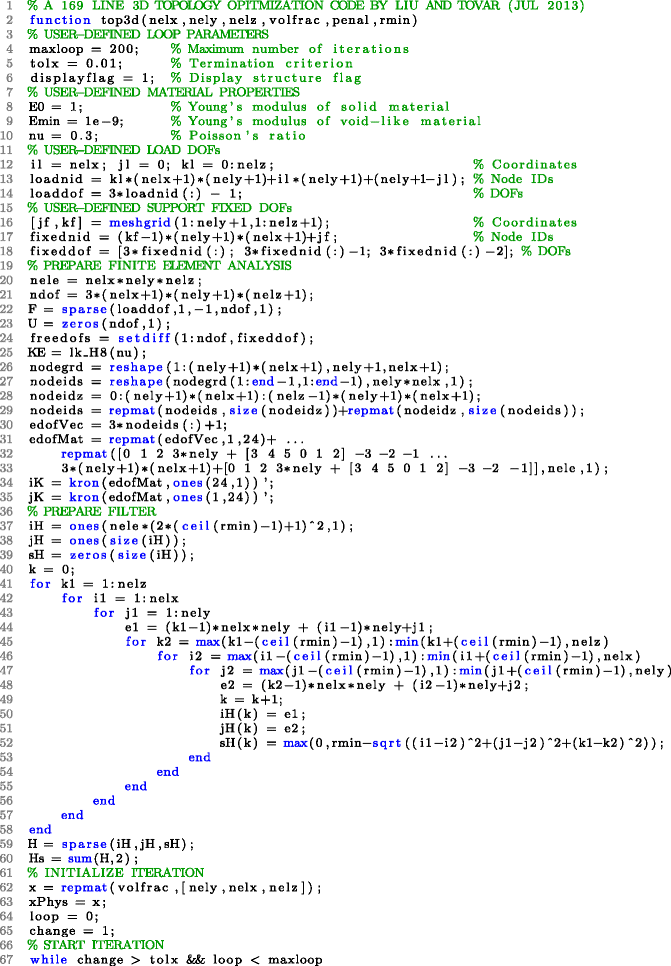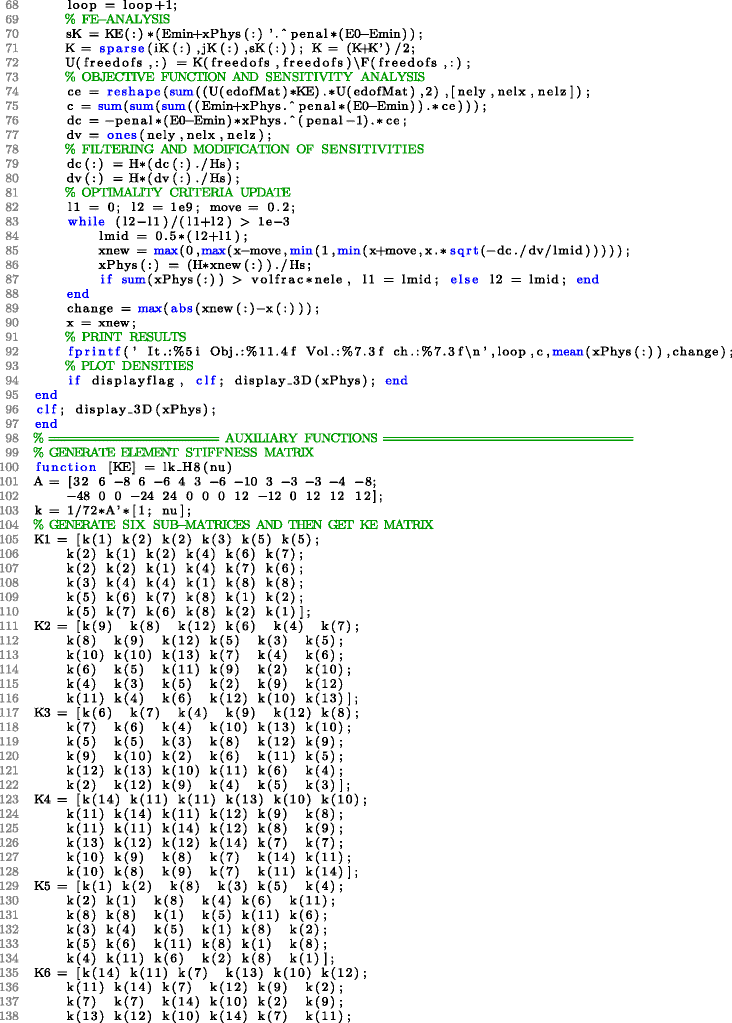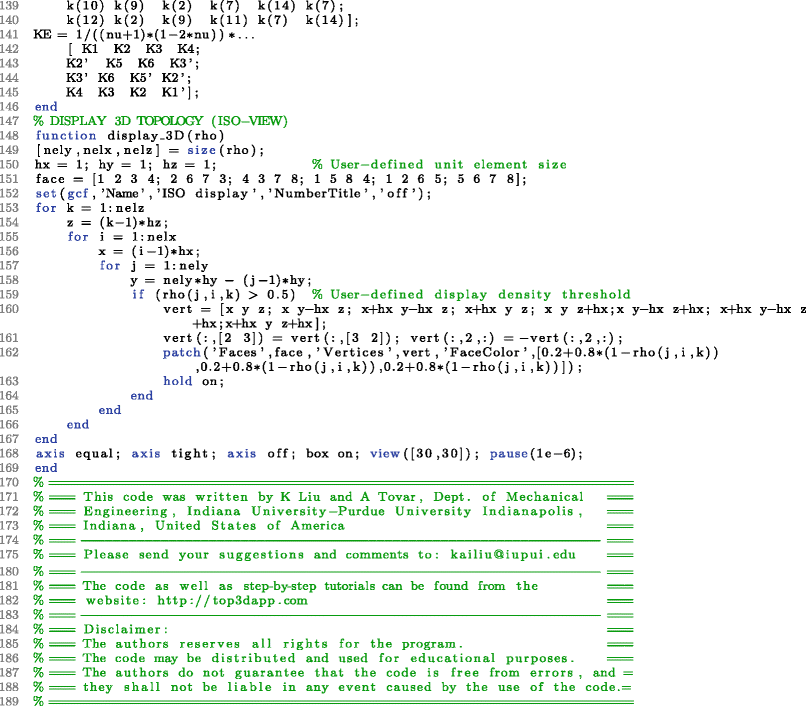## Rights and permissions

Open Access This article is distributed under the terms of the Creative Commons Attribution 4.0 International License (https://creativecommons.org/licenses/by/4.0), which permits use, duplication, adaptation, distribution, and reproduction in any medium or format, as long as you give appropriate credit to the original author(s) and the source, provide a link to the Creative Commons license, and indicate if changes were made.

Reprints and Permissions